UIHistories Project: A History of the University of Illinois by Kalev Leetaru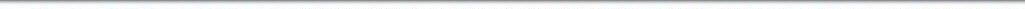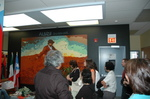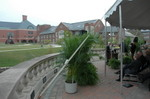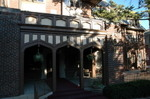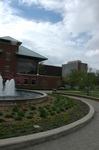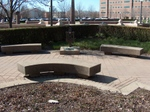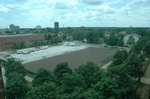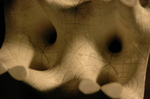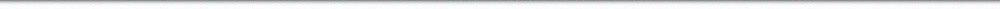N A V I G A T I O N D I G I T A L L I B R A R Y

## Repository: UIHistories Project: Board of Trustees Minutes - 1896 [PAGE 30]

Caption: Board of Trustees Minutes - 1896
This is a reduced-resolution page image for fast online browsing.

< Previous Page [Displaying Page 30 of 371] Next Page >
[VIEW ALL PAGE THUMBNAILS]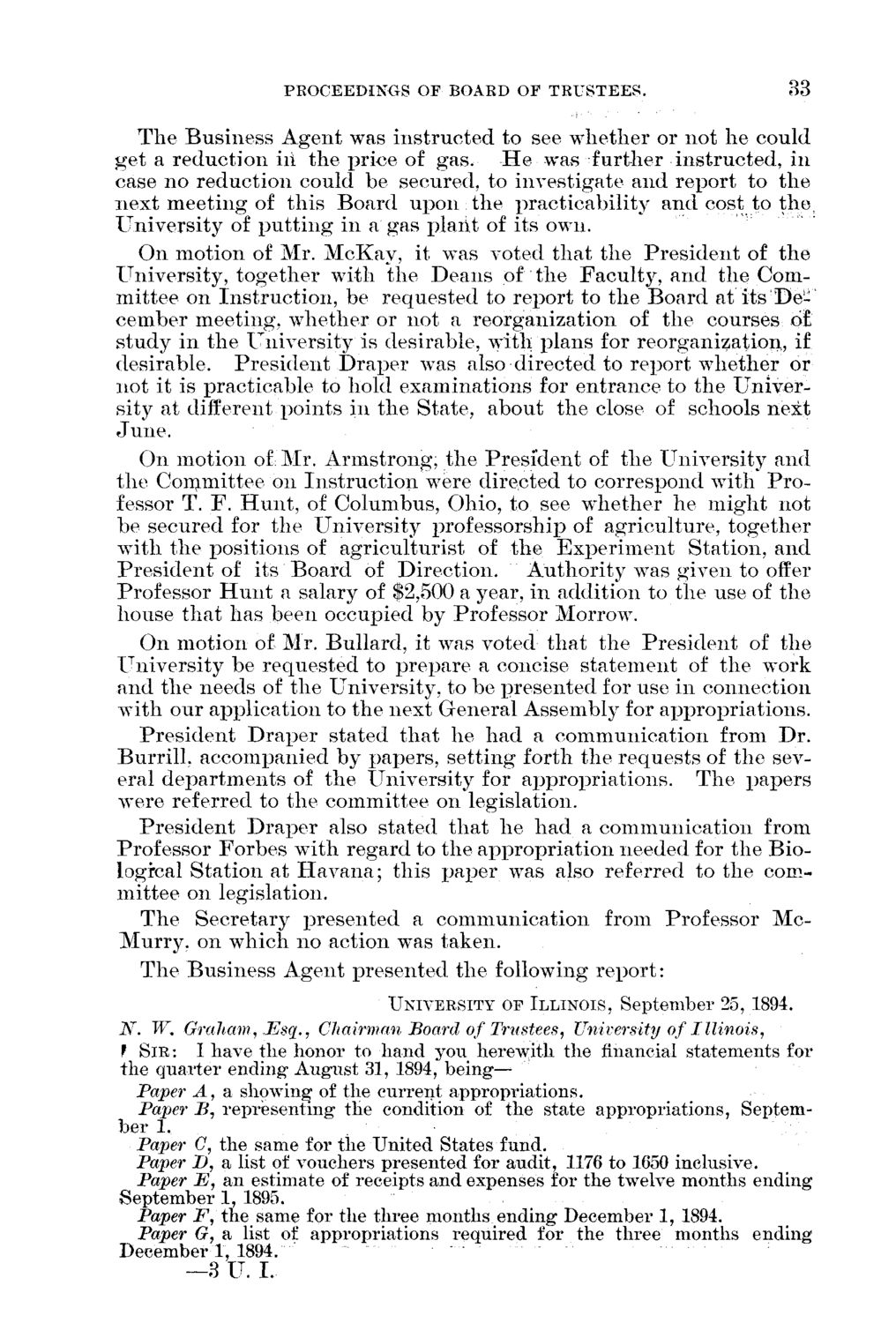### EXTRACTED TEXT FROM PAGE:

PROCEEDINGS OF BOARD OF TRUSTEES.

33

T h e B u s i n e s s A g e n t was i n s t r u c t e d to see w h e t h e r or not h e could g e t a r e d u c t i o n ill t h e r^rice of gas. H e was further i n s t r u c t e d , in case n o r e d u c t i o n could be secured, to investigate a n d r e p o r t to t h e n e x t m e e t i n g of t h i s B o a r d u p o n . t h e p r a c t i c a b i l i t y a n d cost to the, U n i v e r s i t y of p u t t i n g in a gas p l a n t of its own. O n m o t i o n of Mr. M c K a y , it was voted t h a t t h e P r e s i d e n t of t h e U n i v e r s i t y , t o g e t h e r with t h e D e a n s of t h e F a c u l t y , a n d t h e Comm i t t e e on I n s t r u c t i o n , be r e q u e s t e d to r e p o r t to t h e B o a r d at its D e c e m b e r m e e t i n g , w h e t h e r or n o t a reorganization of t h e courses of; s t u d y in t h e U n i v e r s i t y is desirable, with p l a n s for reorganization, if desirable. P r e s i d e n t D r a p e r was also directed to r e p o r t w h e t h e r or n o t it is practicable to hold e x a m i n a t i o n s for e n t r a n c e to t h e U n i v e r sity at different p o i n t s in t h e S t a t e , a b o u t t h e close of schools n e x t June. O n m o t i o n of Mr. A r m s t r o n g , t h e P r e s i d e n t of t h e U n i v e r s i t y a n d t h e C o m m i t t e e on I n s t r u c t i o n were directed to correspond w i t h P r o fessor T. F . H u n t , of C o l u m b u s , Ohio, to see w r hether he m i g h t not b e secured for t h e U n i v e r s i t y professorship of a g r i c u l t u r e , t o g e t h e r w i t h t h e positions of a g r i c u l t u r i s t of t h e E x p e r i m e n t S t a t i o n , a n d P r e s i d e n t of its B o a r d of D i r e c t i o n . A u t h o r i t y was given to offer Professor H u n t a salary of \$2,500 a year, in addition to t h e use of t h e house t h a t has been occupied by Professor Morrow. O n m o t i o n of Mr. B u l l a r d , it was voted t h a t t h e P r e s i d e n t of t h e U n i v e r s i t y be r e q u e s t e d to p r e p a r e a concise s t a t e m e n t of t h e work a n d t h e needs of t h e U n i v e r s i t y , to be p r e s e n t e d for use in connection w i t h our application to t h e next G e n e r a l Assembly for a p p r o p r i a t i o n s . P r e s i d e n t D r a p e r stated t h a t h e h a d a c o m m u n i c a t i o n from D r . B u r r i l l , accompanied by papers, s e t t i n g forth t h e r e q u e s t s of t h e several d e p a r t m e n t s of t h e U n i v e r s i t y for a p p r o p r i a t i o n s . T h e p a p e r s were referred to t h e committee on legislation. P r e s i d e n t Drarjer also stated t h a t h e h a d a c o m m u n i c a t i o n from Professor F o r b e s w i t h regard to t h e a p p r o p r i a t i o n needed for t h e Biologrcal S t a t i o n at H a v a n a ; t h i s p a p e r was also referred to t h e comm i t t e e on legislation. T h e S e c r e t a r y p r e s e n t e d a c o m m u n i c a t i o n from Professor M c M u r r y . on w h i c h n o action was taken. T h e B u s i n e s s A g e n t p r e s e n t e d t h e following r e p o r t :

UNIVERSITY OF ILLINOIS, September 25, 1894.

N. W* Graham, Esq., Chairman Board of Trustees, University of Illinois, f SIR : I have the honor to hand you herewith the financial statements for the quarter ending* August 31, 1894, b e i n g Paper A, a showing of the current appropriations. Paper B, representing the condition of the state appropriations, September 1. Paper C, the same for the United States fund. Paper D, a list of vouchers presented for audit, 1176 to 1650 inclusive. Paper E, an estimate of receipts and expenses for the twelve months ending September 1, 1895. Paper F, the same for the three months ending December 1, 1894. Paper G, a list of appropriations required for the three months ending December 1, 1894. —3 U. I.